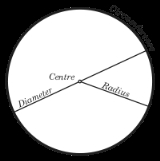xCircleWordNet

### noun

(1)   Movement once around a course
"He drove an extra lap just for insurance"
(2)   Any circular or rotating mechanism
"The machine punched out metal circles"
(3)   A curved section or tier of seats in a hall or theater or opera house; usually the first tier above the orchestra
"They had excellent seats in the dress circle"
(4)   Street names for flunitrazepan
(5)   A road junction at which traffic streams circularly around a central island
"The accident blocked all traffic at the rotary"
(6)   An unofficial association of people or groups
"The smart set goes there"
"They were an angry lot"
(7)   Ellipse in which the two axes are of equal length; a plane curve generated by one point moving at a constant distance from a fixed point
"He calculated the circumference of the circle"
(8)   Something approximating the shape of a circle
"The chairs were arranged in a circle"

### verb

(9)   Travel around something
"Circle the globe"
(10)   Move in circles
(11)   Be around
"Developments surround the town"
"The river encircles the village"WiktionaryText

### Noun

1. : A two-dimensional geometric figure, a line, consisting of the set of all those points in a plane that are equally distant from another point.
The set of all points (x, y) such that $\left(x-1\right)^2 + y^2 = r^2$ is a circle of radius r around the point (1, 0).
2. A two-dimensional geometric figure, a disk, consisting of the set of all those points of a plane at a distance less than or equal to a fixed distance from another point.
3. Any thin three-dimensional equivalent of the geometric figures.
Put on your dunce-cap and sit down on that circle.
4. A curve that more or less forms part or all of a circle.
move in a circle
5. Orbit.
6. A specific group of persons.
inner circle
circle of friends
7. A line comprising two semicircles of 30 yards radius centred on the wickets joined by straight lines parallel to the pitch used to enforce field restrictions in a one-day match.

#### Synonyms

• (two-dimensional outline geometric figure): coil (not in mathematical use), ring (not in mathematical use), loop (not in mathematical use)
• (two-dimensional solid geometric figure): disc/disk (in mathematical and general use), round (not in mathematical use; UK & Commonwealth only)
• (curve): arc, curve
• (orbit): orbit

### Verb

1. To travel around along a curved path.
2. To surround.
3. To place or mark a circle around.
Circle the jobs that you are interested in applying for.
4. To travel in circles.
Vultures circled overhead.